This book is archived and will be removed July 6, 2022. Please use the updated version.

Chapter 15. Equilibria of Other Reaction Classes

# 15.1 Precipitation and Dissolution

### Learning Objectives

By the end of this section, you will be able to:
• Write chemical equations and equilibrium expressions representing solubility equilibria
• Carry out equilibrium computations involving solubility, equilibrium expressions, and solute concentrations

The preservation of medical laboratory blood samples, mining of sea water for magnesium, formulation of over-the-counter medicines such as Milk of Magnesia and antacids, and treating the presence of hard water in your home’s water supply are just a few of the many tasks that involve controlling the equilibrium between a slightly soluble ionic solid and an aqueous solution of its ions.

In some cases, we want to prevent dissolution from occurring. Tooth decay, for example, occurs when the calcium hydroxylapatite, which has the formula Ca5(PO4)3(OH), in our teeth dissolves. The dissolution process is aided when bacteria in our mouths feast on the sugars in our diets to produce lactic acid, which reacts with the hydroxide ions in the calcium hydroxylapatite. Preventing the dissolution prevents the decay. On the other hand, sometimes we want a substance to dissolve. We want the calcium carbonate in a chewable antacid to dissolve because the $\text{CO}_3^{\;\;2-}$ ions produced in this process help soothe an upset stomach.

In this section, we will find out how we can control the dissolution of a slightly soluble ionic solid by the application of Le Châtelier’s principle. We will also learn how to use the equilibrium constant of the reaction to determine the concentration of ions present in a solution.

# The Solubility Product Constant

Silver chloride is what’s known as a sparingly soluble ionic solid (Figure 1). Recall from the solubility rules in an earlier chapter that halides of Ag+ are not normally soluble. However, when we add an excess of solid AgCl to water, it dissolves to a small extent and produces a mixture consisting of a very dilute solution of Ag+ and Cl ions in equilibrium with undissolved silver chloride:

$\text{AgCl}(s)\;\underset{\text{precipitation}}{\overset{\text{dissolution}}{\rightleftharpoons}}\;\text{Ag}^{+}(aq)\;+\;\text{Cl}^{-}(aq)$

This equilibrium, like other equilibria, is dynamic; some of the solid AgCl continues to dissolve, but at the same time, Ag+ and Cl ions in the solution combine to produce an equal amount of the solid. At equilibrium, the opposing processes have equal rates.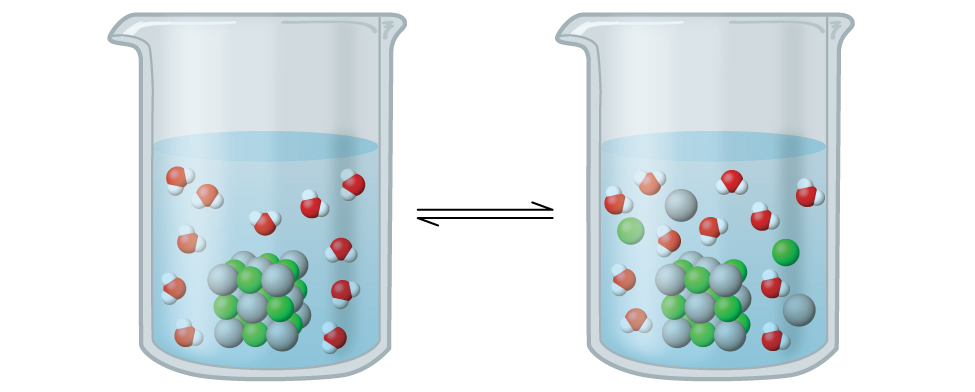Figure 1. Silver chloride is a sparingly soluble ionic solid. When it is added to water, it dissolves slightly and produces a mixture consisting of a very dilute solution of Ag+ and Cl– ions in equilibrium with undissolved silver chloride.

The equilibrium constant for the equilibrium between a slightly soluble ionic solid and a solution of its ions is called the solubility product (Ksp) of the solid. Recall from the chapter on solutions and colloids that we use an ion’s concentration as an approximation of its activity in a dilute solution. For silver chloride, at equilibrium:

$\text{AgCl}(s)\;{\rightleftharpoons}\;\text{Ag}^{+}(aq)\;+\;\text{Cl}^{-}(aq)\;\;\;\;\;\;\;K_{\text{sp}} = [\text{Ag}^{+}(aq)][\text{Cl}^{-}(aq)]$

When looking at dissolution reactions such as this, the solid is listed as a reactant, whereas the ions are listed as products. The solubility product constant, as with every equilibrium constant expression, is written as the product of the concentrations of each of the ions, raised to the power of their stoichiometric coefficients. Here, the solubility product constant is equal to Ag+ and Cl when a solution of silver chloride is in equilibrium with undissolved AgCl. There is no denominator representing the reactants in this equilibrium expression since the reactant is a pure solid; therefore [AgCl] does not appear in the expression for Ksp.

Some common solubility products are listed in Table 1 according to their Ksp values, whereas a more extensive compilation of products appears in Appendix J. Each of these equilibrium constants is much smaller than 1 because the compounds listed are only slightly soluble. A small Ksp represents a system in which the equilibrium lies to the left, so that relatively few hydrated ions would be present in a saturated solution.

Substance Ksp at 25 °C
CuCl 1.2 × 10–6
CuBr 6.27 × 10–9
AgI 1.5 × 10–16
PbS 7 × 10–29
Al(OH)3 2 × 10–32
Fe(OH)3 4 × 10–38
Table 1. Common Solubility Products by Decreasing Equilibrium Constants

### Example 1

Writing Equations and Solubility Products
Write the ionic equation for the dissolution and the solubility product expression for each of the following slightly soluble ionic compounds:

(a) AgI, silver iodide, a solid with antiseptic properties

(b) CaCO3, calcium carbonate, the active ingredient in many over-the-counter chewable antacids

(c) Mg(OH)2, magnesium hydroxide, the active ingredient in Milk of Magnesia

(d) Mg(NH4)PO4, magnesium ammonium phosphate, an essentially insoluble substance used in tests for magnesium

(e) Ca5(PO4)3OH, the mineral apatite, a source of phosphate for fertilizers

(Hint: When determining how to break (d) and (e) up into ions, refer to the list of polyatomic ions in the section on chemical nomenclature.)

Solution
(a) $\text{AgI}(s)\;{\rightleftharpoons}\;\text{Ag}^{+}(aq)\;+\;\text{I}^{-}(aq)\;\;\;\;\;\;\;K_{\text{sp}} = [\text{Ag}^{+}][\text{I}^{-}]$

(b) $\text{CaCO}_3(s)\;{\rightleftharpoons}\;\text{Ca}^{2+}(aq)\;+\;\text{CO}_3^{\;\;2-}(aq)\;\;\;\;\;\;\;K_{\text{sp}} = [\text{Ca}^{2+}][\text{CO}_3^{\;\;2-}]$

(c) $\text{Mg(OH)}_2(s)\;{\rightleftharpoons}\;\text{Mg}^{2+}(aq)\;+\;2\text{OH}^{-}(aq)\;\;\;\;\;\;\;K_{\text{sp}} = [\text{Mg}^{2+}][\text{OH}^{-}]^2$

(d) $\text{Mg(NH}_4)\text{PO}_4(s)\;{\rightleftharpoons}\;\text{Mg}^{2+}(aq)\;+\;\text{NH}_4^{\;\;+}(aq)\;+\;\text{PO}_4^{\;\;3-}(aq)\;\;\;\;\;\;\;K_{\text{sp}} = [\text{Mg}^{2+}][\text{NH}_4^{\;\;+}][\text{PO}_4^{\;\;3-}]$

(e) $\text{Ca}_5(\text{PO}_4)3\text{OH}(s)\;{\rightleftharpoons}\;5\text{Ca}^{2+}(aq)\;+\;3\text{PO}_4^{\;\;3-}(aq)\;+\;\text{OH}^{-}(aq)\;\;\;\;\;\;\;K_{\text{sp}} = [\text{Ca}^{2+}]^5[\text{PO}_4^{\;\;3-}]^3[\text{OH}^{-}]$

Write the ionic equation for the dissolution and the solubility product for each of the following slightly soluble compounds:

(a) BaSO4

(b) Ag2SO4

(c) Al(OH)3

(d) Pb(OH)Cl

(a) $\text{BaSO}_4(s)\;{\rightleftharpoons}\;\text{Ba}^{2+}(aq)\;+\;\text{SO}_4^{\;\;2-}(aq)\;\;\;\;\;\;\;K_{\text{sp}} = [\text{Ba}^{2+}][\text{SO}_4^{\;\;2-}]$; (b) $\text{Ag}_2\text{SO}_4(s)\;{\rightleftharpoons}\;2\text{Ag}^{+}(aq)\;+\;\text{SO}_4^{\;\;2-}(aq)\;\;\;\;\;\;\;K_{\text{sp}} = [\text{Ag}^{+}]^2[\text{SO}_4^{\;\;2-}]$; (c) $\text{Al(OH)}_3(s)\;{\rightleftharpoons}\;\text{Al}^{2+}(aq)\;+\;3\text{OH}^{-}(aq)\;\;\;\;\;\;\;K_{\text{sp}} = [\text{Al}^{3+}][\text{OH}^{-}]^3$; (d) $\text{Pb(OH)Cl}(s)\;{\rightleftharpoons}\;\text{Pb}^{2+}(aq)\;+\;\text{OH}^{-}(aq)\;+\;\text{Cl}^{-}(aq)\;\;\;\;\;\;\;K_{\text{sp}} = [\text{Pb}^{2+}][\text{OH}^{-}][\text{Cl}^{-}]$

Now we will extend the discussion of Ksp and show how the solubility product constant is determined from the solubility of its ions, as well as how Ksp can be used to determine the molar solubility of a substance.

# Ksp and Solubility

Recall that the definition of solubility is the maximum possible concentration of a solute in a solution at a given temperature and pressure. We can determine the solubility product of a slightly soluble solid from that measure of its solubility at a given temperature and pressure, provided that the only significant reaction that occurs when the solid dissolves is its dissociation into solvated ions, that is, the only equilibrium involved is:

$\text{M}_p\text{X}_q(s)\;{\rightleftharpoons}\;p\text{M}^{\text{m+}}(aq)\;+\;q\text{X}^{\text{n-}}(aq)$

In this case, we calculate the solubility product by taking the solid’s solubility expressed in units of moles per liter (mol/L), known as its molar solubility.

### Example 2

Calculation of Ksp from Equilibrium Concentrations
We began the chapter with an informal discussion of how the mineral fluorite (Introduction to Chapter 15) is formed. Fluorite, CaF2, is a slightly soluble solid that dissolves according to the equation:

$\text{CaF}_2(s)\;{\rightleftharpoons}\;\text{Ca}^{2+}(aq)\;+\;2\text{F}^{-}(aq)$

The concentration of Ca2+ in a saturated solution of CaF2 is 2.15 × 10–4M; therefore, that of F is 4.30 × 10–4M, that is, twice the concentration of Ca2+. What is the solubility product of fluorite?

Solution
First, write out the Ksp expression, then substitute in concentrations and solve for Ksp:

$\text{CaF}_2(s)\;{\rightleftharpoons}\;\text{Ca}^{2+}(aq)\;+\;2\text{F}^{-}(aq)$

A saturated solution is a solution at equilibrium with the solid. Thus:

$K_{\text{sp}} = [\text{Ca}^{2+}][\text{F}^{-}]^2 = (2.1\;\times\;10^{-4})(4.2\;\times\;10^{-4})^2 = 3.7\;\times\;10^{-11}$

As with other equilibrium constants, we do not include units with Ksp.

In a saturated solution that is in contact with solid Mg(OH)2, the concentration of Mg2+ is 1.31 × 10–4M. What is the solubility product for Mg(OH)2?

$\text{Mg(OH)}_2(s)\;{\rightleftharpoons}\;\text{Mg}^{2+}(aq)\;+\;2\text{OH}^{-}(aq)$

8.99 × 10–12

### Example 3

Determination of Molar Solubility from Ksp
The Ksp of copper(I) bromide, CuBr, is 6.3 × 10–9. Calculate the molar solubility of copper bromide.

Solution
The solubility product constant of copper(I) bromide is 6.3 × 10–9.

The reaction is:

$\text{CuBr}(s)\;{\rightleftharpoons}\;\text{Cu}^{+}(aq)\;+\;\text{Br}^{-}(aq)$

First, write out the solubility product equilibrium constant expression:

$K_{\text{sp}} = [\text{Cu}^{+}][\text{Br}^{-}]$

Create an ICE table (as introduced in the chapter on fundamental equilibrium concepts), leaving the CuBr column empty as it is a solid and does not contribute to the Ksp: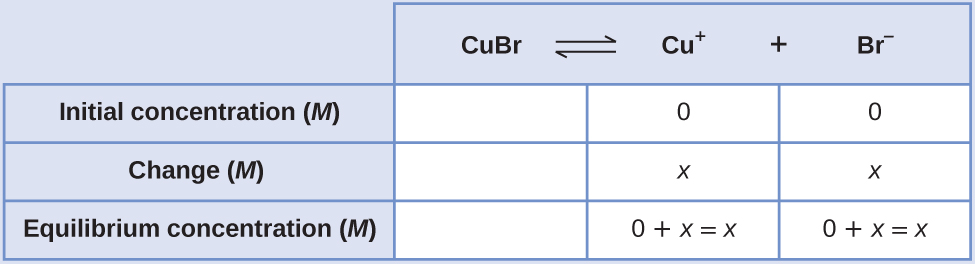At equilibrium:

$K_{\text{sp}} = [\text{Cu}^{+}][\text{Br}^{-}]$
$6.3\;\times\;10^{-9} = (x)(x) = x^2$
$x = \sqrt{(6.3\;\times\;10^{-9})} = 7.9\;\times\;10^{-5}$

Therefore, the molar solubility of CuBr is 7.9 × 10–5M.

The Ksp of AgI is 1.5 × 10–16. Calculate the molar solubility of silver iodide.

1.2 × 10–8 M

### Example 4

Determination of Molar Solubility from Ksp, Part II
The Ksp of calcium hydroxide, Ca(OH)2, is 1.3 × 10–6. Calculate the molar solubility of calcium hydroxide.

Solution
The solubility product constant of calcium hydroxide is 1.3 × 10–6.

The reaction is:

$\text{Ca(OH)}_2(s)\;{\rightleftharpoons}\;\text{Ca}^{2+}(aq)\;+\;2\text{OH}^{-}(aq)$

First, write out the solubility product equilibrium constant expression:

$K_{\text{sp}} = [\text{Ca}^{2+}][\text{OH}^{-}]^2$

Create an ICE table, leaving the Ca(OH)2 column empty as it is a solid and does not contribute to the Ksp: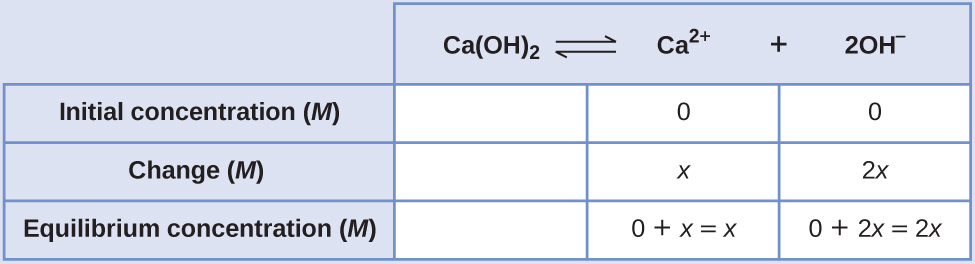At equilibrium:

$K_{\text{sp}} = [\text{Ca}^{2+}][\text{OH}^{-}]^2$
$1.3\;\times\;10^{-6} = (x)(2x)^2 = (x)(4x^2) = 4x^3$
$x = \sqrt{\frac{1.3\;\times\;10^{-6}}{4}} = 6.9\;\times\;10^{-3}$

Therefore, the molar solubility of Ca(OH)2 is 1.3 × 10–2 M.

The Ksp of PbI2 is 1.4 × 10–8. Calculate the molar solubility of lead(II) iodide.

1.5 × 10–3 M

Note that solubility is not always given as a molar value. When the solubility of a compound is given in some unit other than moles per liter, we must convert the solubility into moles per liter (i.e., molarity) in order to use it in the solubility product constant expression. Example 5 shows how to perform those unit conversions before determining the solubility product equilibrium.

### Example 5

Determination of Ksp from Gram Solubility
Many of the pigments used by artists in oil-based paints (Figure 2) are sparingly soluble in water. For example, the solubility of the artist’s pigment chrome yellow, PbCrO4, is 4.6 × 10–6 g/L. Determine the solubility product equilibrium constant for PbCrO4.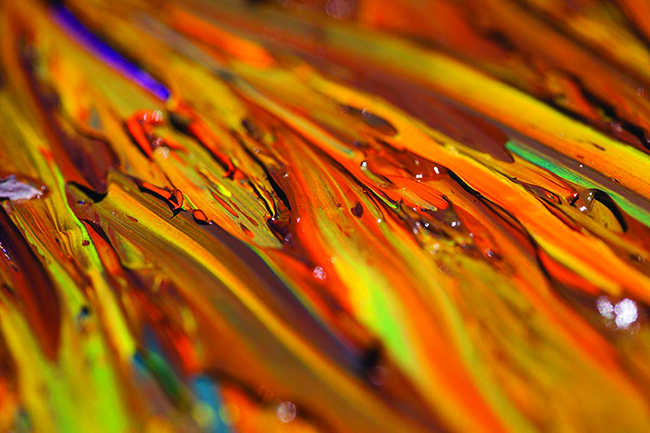Figure 2. Oil paints contain pigments that are very slightly soluble in water. In addition to chrome yellow (PbCrO4), examples include Prussian blue (Fe7(CN)18), the reddish-orange color vermilion (HgS), and green color veridian (Cr2O3). (credit: Sonny Abesamis)

Solution
We are given the solubility of PbCrO4 in grams per liter. If we convert this solubility into moles per liter, we can find the equilibrium concentrations of Pb2+ and $\text{CrO}_4^{\;\;2-}$, then Ksp: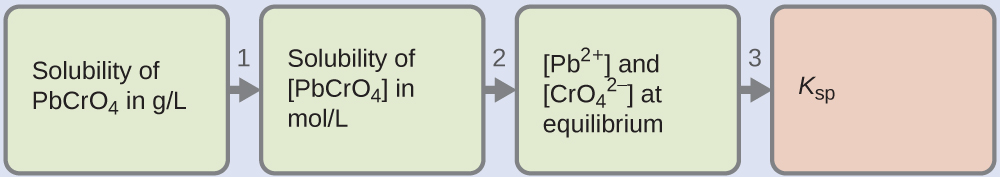1. Use the molar mass of PbCrO4 $(\frac{323.2\;\text{g}}{1\;\text{mol}})$ to convert the solubility of PbCrO4 in grams per liter into moles per liter:
$[\text{PbCrO}_4] = \frac{4.6\;\times\;10^{-6}\;\text{g}\;\text{PbCrO}_4}{1\;\text{L}}\;\times\;\frac{1\;\text{mol}\;\text{PbCrO}_4}{323.2\;\text{g\;PbCrO}_4}$
$= \frac{1.4\;\times\;10^{-8}\;\text{mol\;PbCrO}_4}{1\;\text{L}}$
$= 1.4\;\times\;10^{-8}\;M$
2. The chemical equation for the dissolution indicates that 1 mol of PbCrO4 gives 1 mol of Pb2+(aq) and 1 mol of $\text{CrO}_4^{\;\;2-}(aq)$:
$\text{PbCrO}_4(s)\;{\rightleftharpoons}\;\text{Pb}^{2+}(aq)\;+\;\text{CrO}_4^{\;\;2-}(aq)$

Thus, both [Pb2+] and $[\text{CrO}_4^{\;\;2-}]$ are equal to the molar solubility of PbCrO4:

$[\text{Pb}^{2+}] = [\text{CrO}_4^{\;\;2-}] = 1.4\;\times\;10^{-8}\;M$
3. Solve. Ksp = [Pb2+]$[\text{CrO}_4^{\;\;2-}]$ = (1.4 × 10–8)(1.4 × 10–8) = 2.0 × 10–16

The solubility of TlCl [thallium(I) chloride], an intermediate formed when thallium is being isolated from ores, is 3.46 grams per liter at 20 °C. What is its solubility product?

1.7 × 10–4

### Example 6

Calculating the Solubility of Hg2Cl2
Calomel, Hg2Cl2, is a compound composed of the diatomic ion of mercury(I), $\text{Hg}_2^{\;\;2+}$, and chloride ions, Cl. Although most mercury compounds are now known to be poisonous, eighteenth-century physicians used calomel as a medication. Their patients rarely suffered any mercury poisoning from the treatments because calomel is quite insoluble:

$\text{Hg}_2\text{Cl}_2(s)\;{\rightleftharpoons}\;\text{Hg}_2^{\;\;2+}(aq)\;+\;2\text{Cl}^{-}(aq)\;\;\;\;\;\;\;K_{\text{sp}} = 1.1\;\times\;10^{-18}$

Calculate the molar solubility of Hg2Cl2.

Solution
The molar solubility of Hg2Cl2 is equal to the concentration of $\text{Hg}_2^{\;\;2+}$ ions because for each 1 mol of Hg2Cl2 that dissolves, 1 mol of $\text{Hg}_2^{\;\;2+}$ forms:1. Determine the direction of change. Before any Hg2Cl2 dissolves, Q is zero, and the reaction will shift to the right to reach equilibrium.
2. Determine x and equilibrium concentrations. Concentrations and changes are given in the following ICE table: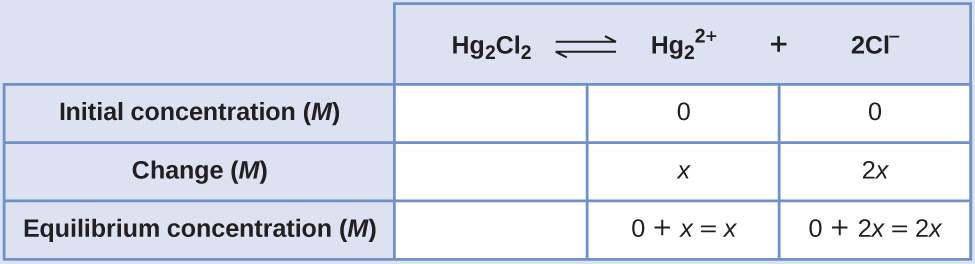Note that the change in the concentration of Cl (2x) is twice as large as the change in the concentration of $\text{Hg}_2^{\;\;2+}$ (x) because 2 mol of Cl forms for each 1 mol of $\text{Hg}_2^{\;\;2+}$ that forms. Hg2Cl2 is a pure solid, so it does not appear in the calculation.

3. Solve for x and the equilibrium concentrations. We substitute the equilibrium concentrations into the expression for Ksp and calculate the value of x:
$K_{\text{sp}} = [\text{Hg}_2^{\;\;2+}][\text{Cl}^{-}]^2$
$1.1\;\times\;10^{-18} = (x)(2x)^2$
$4x^3 = 1.1\;\times\;10^{-18}$
$x = \sqrt{(\frac{1.1\;\times\;10^{-18}}{4})} = 6.5\;\times\;10^{-7}\;M$
$[\text{Hg}_2^{\;\;2+}] = 6.5\;\times\;10^{-7}\;M = 6.5\;\times\;10^{-7}\;M$
$[\text{Cl}^{-}] = 2x = 2(6.5\;\times\;10^{-7}) = 1.3\;\times\;10^{-6}\;M$

The molar solubility of Hg2Cl2 is equal to $[\text{Hg}_2^{\;\;2+}]$, or 6.5 × 10–7M.

4. Check the work. At equilibrium, Q = Ksp:
$Q = [\text{Hg}_2^{\;\;2+}][\text{Cl}^{-}]^2 = (6.5\;\times\;10^{-7})(1.3\;\times\;10^{-6})^2 = 1.1\;\times\;10^{-18}$

The calculations check.

Determine the molar solubility of MgF2 from its solubility product: Ksp = 6.4 × 10–9.

1.2 × 10–3M

Tabulated Ksp values can also be compared to reaction quotients calculated from experimental data to tell whether a solid will precipitate in a reaction under specific conditions: Q equals Ksp at equilibrium; if Q is less than Ksp, the solid will dissolve until Q equals Ksp; if Q is greater than Ksp, precipitation will occur at a given temperature until Q equals Ksp.

### Using Barium Sulfate for Medical Imaging

Various types of medical imaging techniques are used to aid diagnoses of illnesses in a noninvasive manner. One such technique utilizes the ingestion of a barium compound before taking an X-ray image. A suspension of barium sulfate, a chalky powder, is ingested by the patient. Since the Ksp of barium sulfate is 1.1 × 10–10, very little of it dissolves as it coats the lining of the patient’s intestinal tract. Barium-coated areas of the digestive tract then appear on an X-ray as white, allowing for greater visual detail than a traditional X-ray (Figure 3).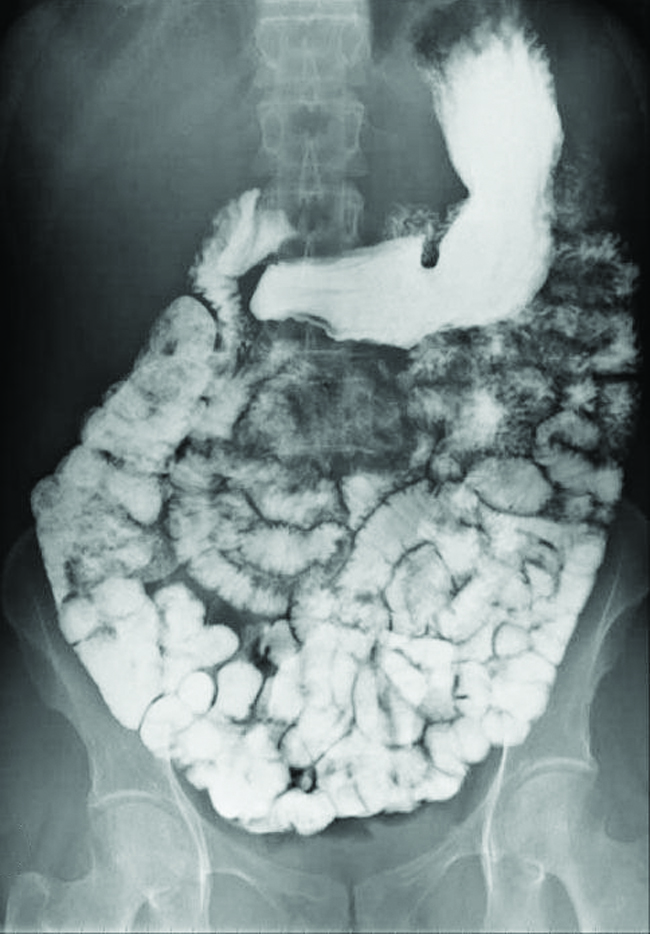Figure 3. The suspension of barium sulfate coats the intestinal tract, which allows for greater visual detail than a traditional X-ray. (credit modification of work by “glitzy queen00”/Wikimedia Commons)

Further diagnostic testing can be done using barium sulfate and fluoroscopy. In fluoroscopy, a continuous X-ray is passed through the body so the doctor can monitor, on a TV or computer screen, the barium sulfate’s movement as it passes through the digestive tract. Medical imaging using barium sulfate can be used to diagnose acid reflux disease, Crohn’s disease, and ulcers in addition to other conditions.

Visit this website for more information on how barium is used in medical diagnoses and which conditions it is used to diagnose.

# Predicting Precipitation

The equation that describes the equilibrium between solid calcium carbonate and its solvated ions is:

$\text{CaCO}_3(s)\;{\rightleftharpoons}\;\text{Ca}^{2+}(aq)\;+\;\text{CO}_3^{\;\;2-}(aq)$

We can establish this equilibrium either by adding solid calcium carbonate to water or by mixing a solution that contains calcium ions with a solution that contains carbonate ions. If we add calcium carbonate to water, the solid will dissolve until the concentrations are such that the value of the reaction quotient $(\text{Q} = [\text{Ca}^{2+}][\text{CO}_3^{\;\;2-}])$ is equal to the solubility product (Ksp = 8.7 × 10–9). If we mix a solution of calcium nitrate, which contains Ca2+ ions, with a solution of sodium carbonate, which contains $\text{CO}_3^{\;\;2-}$ ions, the slightly soluble ionic solid CaCO3 will precipitate, provided that the concentrations of Ca2+ and $\text{CO}_3^{\;\;2-}$ ions are such that Q is greater than Ksp for the mixture. The reaction shifts to the left and the concentrations of the ions are reduced by formation of the solid until the value of Q equals Ksp. A saturated solution in equilibrium with the undissolved solid will result. If the concentrations are such that Q is less than Ksp, then the solution is not saturated and no precipitate will form.

We can compare numerical values of Q with Ksp to predict whether precipitation will occur, as Example 7 shows. (Note: Since all forms of equilibrium constants are temperature dependent, we will assume a room temperature environment going forward in this chapter unless a different temperature value is explicitly specified.)

### Example 7

Precipitation of Mg(OH)2
The first step in the preparation of magnesium metal is the precipitation of Mg(OH)2 from sea water by the addition of lime, Ca(OH)2, a readily available inexpensive source of OH ion:

$\text{Mg(OH)}_2(s)\;{\rightleftharpoons}\;\text{Mg}^{2+}(aq)\;+\;2\text{OH}^{-}(aq)\;\;\;\;\;\;\;K_{\text{sp}} = 8.9\;\times\;10^{-12}$

The concentration of Mg2+(aq) in sea water is 0.0537 M. Will Mg(OH)2 precipitate when enough Ca(OH)2 is added to give a [OH] of 0.0010 M?

Solution
This problem asks whether the reaction:

$\text{Mg(OH)}_2(s)\;{\leftrightharpoons}\;\text{Mg}^{2+}(aq)\;+\;2\text{OH}^{-}(aq)$

shifts to the left and forms solid Mg(OH)2 when [Mg2+] = 0.0537 M and [OH] = 0.0010 M. The reaction shifts to the left if Q is greater than Ksp. Calculation of the reaction quotient under these conditions is shown here:

$Q = [\text{Mg}^{2+}][\text{OH}^{-}]^2 = (0.0537)(0.0010)^2 = 5.4\;\times\;10^{-8}$

Because Q is greater than Ksp (Q = 5.4 × 10–8 is larger than Ksp = 8.9 × 10–12), we can expect the reaction to shift to the left and form solid magnesium hydroxide. Mg(OH)2(s) forms until the concentrations of magnesium ion and hydroxide ion are reduced sufficiently so that the value of Q is equal to Ksp.

Use the solubility product in Appendix J to determine whether CaHPO4 will precipitate from a solution with [Ca2+] = 0.0001 M and $[\text{HPO}_4^{\;\;2-}]$ = 0.001 M.

No precipitation of CaHPO4; Q = 1 × 10–7, which is less than Ksp

### Example 8

Precipitation of AgCl upon Mixing Solutions
Does silver chloride precipitate when equal volumes of a 2.0 × 10–4M solution of AgNO3 and a 2.0 × 10–4M solution of NaCl are mixed?

(Note: The solution also contains Na+ and $\text{NO}_3^{\;\;-}$ ions, but when referring to solubility rules, one can see that sodium nitrate is very soluble and cannot form a precipitate.)

Solution
The equation for the equilibrium between solid silver chloride, silver ion, and chloride ion is:

$\text{AgCl}(s)\;{\rightleftharpoons}\;\text{Ag}^{+}(aq)\;+\;\text{Cl}^{-}(aq)$

The solubility product is 1.6 × 10–10 (see Appendix J).

AgCl will precipitate if the reaction quotient calculated from the concentrations in the mixture of AgNO3 and NaCl is greater than Ksp. The volume doubles when we mix equal volumes of AgNO3 and NaCl solutions, so each concentration is reduced to half its initial value. Consequently, immediately upon mixing, [Ag+] and [Cl] are both equal to:

$\frac{1}{2}(2.0\;\times\;10^{-4})\;M = 1.0\;\times\;10^{-4}\;M$

The reaction quotient, Q, is momentarily greater than Ksp for AgCl, so a supersaturated solution is formed:

$Q = [\text{Ag}^{+}][\text{Cl}^{-}] = (1.0\;\times\;10^{-4})(1.0\;\times\;10^{-4}) = 1.0\;\times\;10^{-8}\;{\textgreater}\;K_{\text{sp}}$

Since supersaturated solutions are unstable, AgCl will precipitate from the mixture until the solution returns to equilibrium, with Q equal to Ksp.

Will KClO4 precipitate when 20 mL of a 0.050-M solution of K+ is added to 80 mL of a 0.50-M solution of $\text{ClO}_4^{\;\;-}$? (Remember to calculate the new concentration of each ion after mixing the solutions before plugging into the reaction quotient expression.)

No, Q = 4.0 × 10–3, which is less than Ksp = 1.05 × 10–2

In the previous two examples, we have seen that Mg(OH)2 or AgCl precipitate when Q is greater than Ksp. In general, when a solution of a soluble salt of the Mm+ ion is mixed with a solution of a soluble salt of the Xn– ion, the solid, MpXq precipitates if the value of Q for the mixture of Mm+ and Xn– is greater than Ksp for MpXq. Thus, if we know the concentration of one of the ions of a slightly soluble ionic solid and the value for the solubility product of the solid, then we can calculate the concentration that the other ion must exceed for precipitation to begin. To simplify the calculation, we will assume that precipitation begins when the reaction quotient becomes equal to the solubility product constant.

### Example 9

Precipitation of Calcium Oxalate
Blood will not clot if calcium ions are removed from its plasma. Some blood collection tubes contain salts of the oxalate ion, $\text{C}_2\text{O}_4^{\;\;2-}$, for this purpose (Figure 4). At sufficiently high concentrations, the calcium and oxalate ions form solid, CaC2O4·H2O (which also contains water bound in the solid). The concentration of Ca2+ in a sample of blood serum is 2.2 × 10–3M. What concentration of $\text{C}_2\text{O}_4^{\;\;2-}$ ion must be established before CaC2O4·H2O begins to precipitate?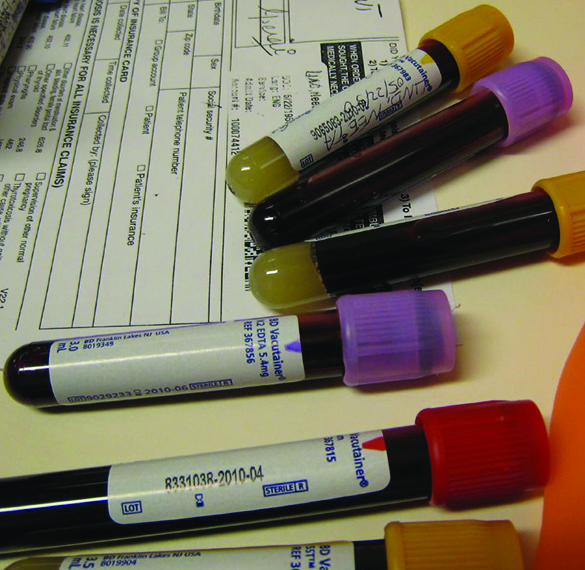Figure 4. Anticoagulants can be added to blood that will combine with the Ca2+ ions in blood serum and prevent the blood from clotting. (credit: modification of work by Neeta Lind)

Solution
The equilibrium expression is:

$\text{CaC}_2\text{O}_4(s)\;{\rightleftharpoons}\;\text{Ca}^{2+}(aq)\;+\;\text{C}_2\text{O}_4^{\;\;2-}(aq)$

For this reaction:

$K_{\text{sp}} = [\text{Ca}^{2+}][\text{C}_2\text{O}_4^{\;\;2-}] = 1.96\;\times\;10^{-8}$

(see Appendix J)

CaC2O4 does not appear in this expression because it is a solid. Water does not appear because it is the solvent.

Solid CaC2O4 does not begin to form until Q equals Ksp. Because we know Ksp and [Ca2+], we can solve for the concentration of $\text{C}_2\text{O}_4^{\;\;2-}$ that is necessary to produce the first trace of solid:

$Q = K_{\text{sp}} = [\text{Ca}^{2+}][\text{C}_2\text{O}_4^{\;\;2-}] = 1.96\;\times\;10^{-8}$
$(2.2\;\times\;10^{-3})[\text{C}_2\text{O}_4^{\;\;2-}] = 1.96\;\times\;10^{-8}$
$[\text{C}_2\text{O}_4^{\;\;2-}] = \frac{1.96\;\times\;10^{-8}}{2.2\;\times\;10^{-3}} = 8.9\;\times\;10^{-6}$

A concentration of $[\text{C}_2\text{O}_4^{\;\;2-}]$ = 8.9 × 10–6 M is necessary to initiate the precipitation of CaC2O4 under these conditions.

If a solution contains 0.0020 mol of $\text{CrO}_4^{\;\;2-}$ per liter, what concentration of Ag+ ion must be reached by adding solid AgNO3 before Ag2CrO4 begins to precipitate? Neglect any increase in volume upon adding the solid silver nitrate.

4.5 × 10–9M

It is sometimes useful to know the concentration of an ion that remains in solution after precipitation. We can use the solubility product for this calculation too: If we know the value of Ksp and the concentration of one ion in solution, we can calculate the concentration of the second ion remaining in solution. The calculation is of the same type as that in Example 9—calculation of the concentration of a species in an equilibrium mixture from the concentrations of the other species and the equilibrium constant. However, the concentrations are different; we are calculating concentrations after precipitation is complete, rather than at the start of precipitation.

### Example 10

Concentrations Following Precipitation
Clothing washed in water that has a manganese [Mn2+(aq)] concentration exceeding 0.1 mg/L (1.8 × 10–6M) may be stained by the manganese upon oxidation, but the amount of Mn2+ in the water can be reduced by adding a base. If a person doing laundry wishes to add a buffer to keep the pH high enough to precipitate the manganese as the hydroxide, Mn(OH)2, what pH is required to keep [Mn2+] equal to 1.8 × 10–6M?

Solution
The dissolution of Mn(OH)2 is described by the equation:

$\text{Mn(OH)}_2(s)\;{\rightleftharpoons}\;\text{Mn}^{2+}(aq)\;+\;2\text{OH}^{-}(aq)\;\;\;\;\;\;\;K_{\text{sp}} = 2\;\times\;10^{-3}$

We need to calculate the concentration of OH when the concentration of Mn2+ is 1.8 × 10–6M. From that, we calculate the pH. At equilibrium:

$K_{\text{sp}} = [\text{Mn}^{2+}][\text{OH}^{-}]^2$

or

$(1.8\;\times\;10^{-6})[\text{OH}^{-}]^2 = 2\;\times\;10^{-3}$

so

$[\text{OH}^{-}] = 3.3\;\times\;10^{-4}\;M$

Now we calculate the pH from the pOH:

$\text{pOH} = -\text{log}[\text{OH}^{-}] = -\text{log}(3.3\;\times\;10\;-\;4) = 3.48$
$\text{pH} = 14.00\;-\;\text{pOH} = 14.00\;-\;3.80 = 10.52$

If the person doing laundry adds a base, such as the sodium silicate (Na4SiO4) in some detergents, to the wash water until the pH is raised to 10.52, the manganese ion will be reduced to a concentration of 1.8 × 10–6M; at that concentration or less, the ion will not stain clothing.

The first step in the preparation of magnesium metal is the precipitation of Mg(OH)2 from sea water by the addition of Ca(OH)2. The concentration of Mg2+(aq) in sea water is 5.37 × 10–2M. Calculate the pH at which [Mg2+] is diminished to 1.0 × 10–5M by the addition of Ca(OH)2.

10.97

Due to their light sensitivity, mixtures of silver halides are used in fiber optics for medical lasers, in photochromic eyeglass lenses (glass lenses that automatically darken when exposed to sunlight), and—before the advent of digital photography—in photographic film. Even though AgCl (Ksp = 1.6 × 10–10), AgBr (Ksp = 5.0 × 10–13), and AgI (Ksp = 1.5 × 10–16) are each quite insoluble, we cannot prepare a homogeneous solid mixture of them by adding Ag+ to a solution of Cl, Br, and I; essentially all of the AgI will precipitate before any of the other solid halides form because of its smaller value for Ksp. However, we can prepare a homogeneous mixture of the solids by slowly adding a solution of Cl, Br, and I to a solution of Ag+.

When two anions form slightly soluble compounds with the same cation, or when two cations form slightly soluble compounds with the same anion, the less soluble compound (usually, the compound with the smaller Ksp) generally precipitates first when we add a precipitating agent to a solution containing both anions (or both cations). When the Ksp values of the two compounds differ by two orders of magnitude or more (e.g., 10–2 vs. 10–4), almost all of the less soluble compound precipitates before any of the more soluble one does. This is an example of selective precipitation, where a reagent is added to a solution of dissolved ions causing one of the ions to precipitate out before the rest.

### The Role of Precipitation in Wastewater Treatment

Solubility equilibria are useful tools in the treatment of wastewater carried out in facilities that may treat the municipal water in your city or town (Figure 5). Specifically, selective precipitation is used to remove contaminants from wastewater before it is released back into natural bodies of water. For example, phosphate ions $(\text{PO}_4^{\;\;2-})$ are often present in the water discharged from manufacturing facilities. An abundance of phosphate causes excess algae to grow, which impacts the amount of oxygen available for marine life as well as making water unsuitable for human consumption.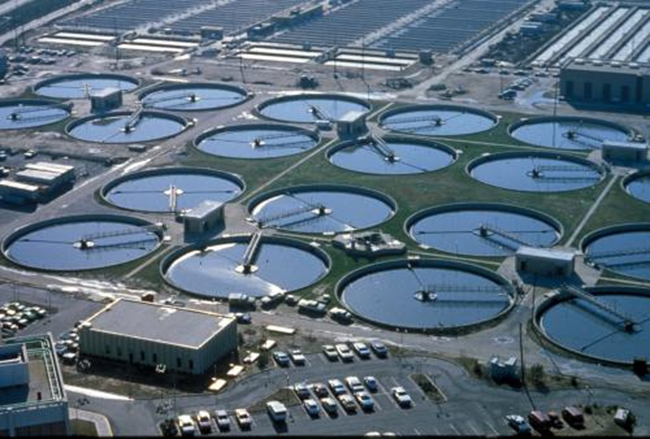Figure 5. Wastewater treatment facilities, such as this one, remove contaminants from wastewater before the water is released back into the natural environment. (credit: “eutrophication&hypoxia”/Wikimedia Commons)

One common way to remove phosphates from water is by the addition of calcium hydroxide, known as lime, Ca(OH)2. The lime is converted into calcium carbonate, a strong base, in the water. As the water is made more basic, the calcium ions react with phosphate ions to produce hydroxylapatite, Ca5(PO4)3(OH), which then precipitates out of the solution:

$5\text{Ca}^{2+}\;+\;3\text{PO}_4^{\;\;3-}\;+\;\text{OH}^{-}\;{\leftrightharpoons}\;\text{Ca}_{10}(\text{PO}_4)_6{\cdot}(\text{OH})_2(s)$

The precipitate is then removed by filtration and the water is brought back to a neutral pH by the addition of CO2 in a recarbonation process. Other chemicals can also be used for the removal of phosphates by precipitation, including iron(III) chloride and aluminum sulfate.

View this site for more information on how phosphorus is removed from wastewater.

Selective precipitation can also be used in qualitative analysis. In this method, reagents are added to an unknown chemical mixture in order to induce precipitation. Certain reagents cause specific ions to precipitate out; therefore, the addition of the reagent can be used to determine whether the ion is present in the solution.

### Example 11

Precipitation of Silver Halides
A solution contains 0.0010 mol of KI and 0.10 mol of KCl per liter. AgNO3 is gradually added to this solution. Which forms first, solid AgI or solid AgCl?

Solution
The two equilibria involved are:

$\text{AgCl}(s)\;{\rightleftharpoons}\;\text{Ag}^{+}(aq)\;+\;\text{Cl}^{-}(aq)\;\;\;\;\;\;\;K_{\text{sp}} = 1.6\;\times\;10^{-10}$
$\text{AgI}(s)\;{\rightleftharpoons}\;\text{Ag}^{+}(aq)\;+\;\text{I}^{-}(aq)\;\;\;\;\;\;\;K_{\text{sp}} = 1.5\;\times\;10^{-16}$

If the solution contained about equal concentrations of Cl and I, then the silver salt with the smallest Ksp (AgI) would precipitate first. The concentrations are not equal, however, so we should find the [Ag+] at which AgCl begins to precipitate and the [Ag+] at which AgI begins to precipitate. The salt that forms at the lower [Ag+] precipitates first.

For AgI: AgI precipitates when Q equals Ksp for AgI (1.5 × 10–16). When [I] = 0.0010 M:

$Q = [\text{Ag}^{+}][\text{I}^{-}] = [\text{Ag}^{+}](0.0010) = 1.5\;\times\;10^{-16}$
$[\text{Ag}^{+}] = \frac{1.8\;\times\;10^{-10}}{0.10} = 1.6\;\times\;10^{-9}$

AgI begins to precipitate when [Ag+] is 1.5 × 10–13M.

For AgCl: AgCl precipitates when Q equals Ksp for AgCl (1.6 × 10–10). When [Cl] = 0.10 M:

$Q_{\text{sp}} = [\text{Ag}^{+}][\text{Cl}^{-}] = [\text{Ag}^{+}](0.10) = 1.6\;\times\;10^{-10}$
$[\text{Ag}^{+}] = \frac{1.8\;\times\;10^{-10}}{0.10} = 1.6\;\times\;10^{-9}\;M$

AgCl begins to precipitate when [Ag+] is 1.6 × 10–9M.

AgI begins to precipitate at a lower [Ag+] than AgCl, so AgI begins to precipitate first.

If silver nitrate solution is added to a solution which is 0.050 M in both Cl and Br ions, at what [Ag+] would precipitation begin, and what would be the formula of the precipitate?

[Ag+] = 1.0 × 10–11M; AgBr precipitates first

# Common Ion Effect

As we saw when we discussed buffer solutions, the hydronium ion concentration of an aqueous solution of acetic acid decreases when the strong electrolyte sodium acetate, NaCH3CO2, is added. We can explain this effect using Le Châtelier’s principle. The addition of acetate ions causes the equilibrium to shift to the left, decreasing the concentration of $\text{H}_3\text{O}^{+}$ to compensate for the increased acetate ion concentration. This increases the concentration of CH3CO2H:

$\text{CH}_3\text{CO}_2\text{H}\;+\;\text{H}_2\text{O}\;{\rightleftharpoons}\;\text{H}_3\text{O}^{+}\;+\;\text{CH}_3\text{CO}_2^{\;\;-}$

Because sodium acetate and acetic acid have the acetate ion in common, the influence on the equilibrium is called the common ion effect.

The common ion effect can also have a direct effect on solubility equilibria. Suppose we are looking at the reaction where silver iodide is dissolved:

$\text{AgI}(s)\;{\rightleftharpoons}\;\text{Ag}^{+}(aq)\;+\;\text{I}^{-}(aq)$

If we were to add potassium iodide (KI) to this solution, we would be adding a substance that shares a common ion with silver iodide. Le Châtelier’s principle tells us that when a change is made to a system at equilibrium, the reaction will shift to counteract that change. In this example, there would be an excess of iodide ions, so the reaction would shift toward the left, causing more silver iodide to precipitate out of solution.

### Example 12

Common Ion Effect
Calculate the molar solubility of cadmium sulfide (CdS) in a 0.010-M solution of cadmium bromide (CdBr2). The Ksp of CdS is 1.0 × 10–28.

Solution
The first thing you should notice is that the cadmium sulfide is dissolved in a solution that contains cadmium ions. We need to use an ICE table to set up this problem and include the CdBr2 concentration as a contributor of cadmium ions:

$\text{CdS}(s)\;{\leftrightharpoons}\;\text{Cd}^{2+}(aq)\;+\;\text{S}^{2-}(aq)$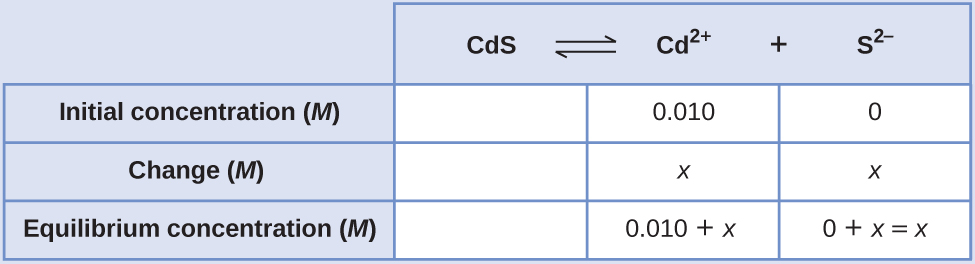$K_{\text{sp}} = [\text{Cd}^{2+}][\text{S}^{2-}] = 1.0\;\times\;10^{-28}$
$(0.010\;+\;x)(x) = 1.0\;\times\;10^{-28}$
$x^2\;+\;0.010x\;-\;1.0\;\times\;10^{-28} = 0$

We can solve this equation using the quadratic formula, but we can also make an assumption to make this calculation much simpler. Since the Ksp value is so small compared with the cadmium concentration, we can assume that the change between the initial concentration and the equilibrium concentration is negligible, so that 0.010 + x ~ 0.010. Going back to our Ksp expression, we would now get:

$K_{\text{sp}} = [\text{Cd}^{2+}][\text{S}^{2-}] = 1.0\;\times\;10^{-28}$
$(0.010)(x) = 1.0\;\times\;10^{-28}$
$x = 1.0\;\times\;10^{-26}$

Therefore, the molar solubility of CdS in this solution is 1.0 × 10–26M.

Calculate the molar solubility of aluminum hydroxide, Al(OH)3, in a 0.015-M solution of aluminum nitrate, Al(NO3)3. The Ksp of Al(OH)3 is 2 × 10–32.

1 × 10–10M

# Key Concepts and Summary

The equilibrium constant for an equilibrium involving the precipitation or dissolution of a slightly soluble ionic solid is called the solubility product, Ksp, of the solid. When we have a heterogeneous equilibrium involving the slightly soluble solid MpXq and its ions Mm+ and Xn–:

$\text{M}_p\text{X}_q(s)\;{\leftrightharpoons}\;p\text{M}^{\text{m}+}(aq)\;+\;q\text{X}^{\text{n}-}(aq)$

We write the solubility product expression as:

$K_{\text{sp}} = [\text{M}^{\text{m}+}]^p[\text{X}^{\text{n}-}]^q$

The solubility product of a slightly soluble electrolyte can be calculated from its solubility; conversely, its solubility can be calculated from its Ksp, provided the only significant reaction that occurs when the solid dissolves is the formation of its ions.

A slightly soluble electrolyte begins to precipitate when the magnitude of the reaction quotient for the dissolution reaction exceeds the magnitude of the solubility product. Precipitation continues until the reaction quotient equals the solubility product.

A reagent can be added to a solution of ions to allow one ion to selectively precipitate out of solution. The common ion effect can also play a role in precipitation reactions. In the presence of an ion in common with one of the ions in the solution, Le Châtelier’s principle applies and more precipitate comes out of solution so that the molar solubility is reduced.

# Key Equations

• $\text{M}_p\text{X}_q(s)\;{\leftrightharpoons}\;p\text{M}^{\text{m}+}(aq)\;+\;q\text{X}^{\text{n}-}(aq)\;\;\;\;\;\;\;K_{\text{sp}} = [\text{M}^{\text{m}+}]^p[\text{X}^{\text{n}-}]^q$

### Chemistry End of Chapter Exercises

1. Complete the changes in concentrations for each of the following reactions:

(a) $\begin{array}{lccc} \text{AgI}(s)\;{\longrightarrow}\; & \text{Ag}^{+}(aq) & + & \text{I}^{-}(aq) \\[0.5em] & x & & \rule[0ex]{2.5em}{0.1ex} \end{array}$

(b) $\begin{array}{lccc} \text{CaCO}_3(s)\;{\longrightarrow} & \text{Ca}^{2+}(aq) & + & \text{CO}_3^{\;\;2-}(aq) \\[0.5em] & \rule[0ex]{2.5em}{0.1ex} & & x \end{array}$

(c) $\begin{array}{lccc} \text{Mg(OH)}_2(s)\;{\longrightarrow} & \text{Mg}^{2+}(aq) & + & 2\text{OH}^{-}(aq) \\[0.5em] & x & & \rule[0ex]{2.5em}{0.1ex} \end{array}$

(d) $\begin{array}{lccc} \text{Mg}_3(\text{PO}_4)_2(s)\;{\longrightarrow} & 3\text{Mg}^{2+}(aq) & + & 2\text{PO}_4^{\;\;3-}(aq) \\[0.5em] & \rule[0ex]{2.5em}{0.1ex} & & 2x \end{array}$

(e) $\begin{array}{lccccc} \text{Ca}_5(\text{PO}_4)_3\text{OH}(s)\;{\longrightarrow} & 5\text{Ca}^{2+}(aq) & + & 3\text{PO}_4^{\;\;3-}(aq) & + & \text{OH}^{-}(aq) \\[0.5em] & \rule[0ex]{2.5em}{0.1ex} & & \rule[0ex]{2.5em}{0.1ex} & & x \end{array}$

2. Complete the changes in concentrations for each of the following reactions:

(a) $\begin{array}{lccc} \text{BaSO}_4(s)\;{\longrightarrow} & \text{Ba}^{2+}(aq) & + & \text{SO}_4^{\;\;2-}(aq) \\[0.5em] & x & & \rule[0ex]{2.5em}{0.1ex} \end{array}$

(b) $\begin{array}{lccc} \text{Ag}_2\text{SO}_4(s)\;{\longrightarrow} & 2\text{Ag}^{+}(aq) & + & \text{SO}_4^{\;\;2-}(aq) \\[0.5em] & \rule[0ex]{2.5em}{0.1ex} & & x \end{array}$

(c) $\begin{array}{lccc} \text{Al(OH)}_3(s)\;{\longrightarrow} & \text{Al}^{3+}(aq) & + & 3\text{OH}^{-}(aq) \\[0.5em] & x & & \rule[0ex]{2.5em}{0.1ex} \end{array}$

(d) $\begin{array}{lccccc} \text{Pb(OH)Cl}(s)\;{\longrightarrow} & \text{Pb}^{2+}(aq) & + & \text{OH}^{-}(aq) & + & \text{Cl}^{-}(aq) \\[0.5em] & \rule[0ex]{2.5em}{0.1ex} & & x & & \rule[0ex]{2.5em}{0.1ex} \end{array}$

(e) $\begin{array}{lccc} \text{Ca}_3(\text{AsO}_4)_2(s)\;{\longrightarrow} & 3\text{Ca}^{2+}(aq) & + & 2\text{AsO}_4^{\;\;3-}(aq) \\[0.5em] & 3x & & \rule[0ex]{2.5em}{0.1ex} \end{array}$

3. How do the concentrations of Ag+ and $\text{CrO}_4^{\;\;2-}$ in a saturated solution above 1.0 g of solid Ag2CrO4 change when 100 g of solid Ag2CrO4 is added to the system? Explain.
4. How do the concentrations of Pb2+ and S2– change when K2S is added to a saturated solution of PbS?
5. What additional information do we need to answer the following question: How is the equilibrium of solid silver bromide with a saturated solution of its ions affected when the temperature is raised?
6. Which of the following slightly soluble compounds has a solubility greater than that calculated from its solubility product because of hydrolysis of the anion present: CoSO3, CuI, PbCO3, PbCl2, Tl2S, KClO4?
7. Which of the following slightly soluble compounds has a solubility greater than that calculated from its solubility product because of hydrolysis of the anion present: AgCl, BaSO4, CaF2, Hg2I2, MnCO3, ZnS, PbS?
8. Write the ionic equation for dissolution and the solubility product (Ksp) expression for each of the following slightly soluble ionic compounds:

(a) PbCl2

(b) Ag2S

(c) Sr3(PO4)2

(d) SrSO4

9. Write the ionic equation for the dissolution and the Ksp expression for each of the following slightly soluble ionic compounds:

(a) LaF3

(b) CaCO3

(c) Ag2SO4

(d) Pb(OH)2

10. The Handbook of Chemistry and Physics gives solubilities of the following compounds in grams per 100 mL of water. Because these compounds are only slightly soluble, assume that the volume does not change on dissolution and calculate the solubility product for each.

(a) BaSiF6, 0.026 g/100 mL (contains $\text{SiF}_6^{\;\;2-}$ ions)

(b) Ce(IO3)4, 1.5 × 10–2 g/100 mL

(c) Gd2(SO4)3, 3.98 g/100 mL

(d) (NH4)2PtBr6, 0.59 g/100 mL (contains $\text{PtBr}_6^{\;\;2-}$ ions)

11. The Handbook of Chemistry and Physics gives solubilities of the following compounds in grams per 100 mL of water. Because these compounds are only slightly soluble, assume that the volume does not change on dissolution and calculate the solubility product for each.

(a) BaSeO4, 0.0118 g/100 mL

(b) Ba(BrO3)2·H2O, 0.30 g/100 mL

(c) NH4MgAsO4·6H2O, 0.038 g/100 mL

(d) La2(MoO4)3, 0.00179 g/100 mL

12. Use solubility products and predict which of the following salts is the most soluble, in terms of moles per liter, in pure water: CaF2, Hg2Cl2, PbI2, or Sn(OH)2.
13. Assuming that no equilibria other than dissolution are involved, calculate the molar solubility of each of the following from its solubility product:

(a) KHC4H4O6

(b) PbI2

(c) Ag4[Fe(CN)6], a salt containing the $\text{Fe(CN)}_4^{\;\;-}$ ion

(d) Hg2I2

14. Assuming that no equilibria other than dissolution are involved, calculate the molar solubility of each of the following from its solubility product:

(a) Ag2SO4

(b) PbBr2

(c) AgI

(d) CaC2O4·H2O

15. Assuming that no equilibria other than dissolution are involved, calculate the concentration of all solute species in each of the following solutions of salts in contact with a solution containing a common ion. Show that changes in the initial concentrations of the common ions can be neglected.

(a) AgCl(s) in 0.025 M NaCl

(b) CaF2(s) in 0.00133 M KF

(c) Ag2SO4(s) in 0.500 L of a solution containing 19.50 g of K2SO4

(d) Zn(OH)2(s) in a solution buffered at a pH of 11.45

16. Assuming that no equilibria other than dissolution are involved, calculate the concentration of all solute species in each of the following solutions of salts in contact with a solution containing a common ion. Show that changes in the initial concentrations of the common ions can be neglected.

(a) TlCl(s) in 1.250 M HCl

(b) PbI2(s) in 0.0355 M CaI2

(c) Ag2CrO4(s) in 0.225 L of a solution containing 0.856 g of K2CrO4

(d) Cd(OH)2(s) in a solution buffered at a pH of 10.995

17. Assuming that no equilibria other than dissolution are involved, calculate the concentration of all solute species in each of the following solutions of salts in contact with a solution containing a common ion. Show that it is not appropriate to neglect the changes in the initial concentrations of the common ions.

(a) TlCl(s) in 0.025 M TlNO3

(b) BaF2(s) in 0.0313 M KF

(c) MgC2O4 in 2.250 L of a solution containing 8.156 g of Mg(NO3)2

(d) Ca(OH)2(s) in an unbuffered solution initially with a pH of 12.700

18. Explain why the changes in concentrations of the common ions in Chapter 15.1 Chemistry End of Chapter Exercise 17 can be neglected.
19. Explain why the changes in concentrations of the common ions in Chapter 15.1 Chemistry End of Chapter Exercise 17 cannot be neglected.
20. Calculate the solubility of aluminum hydroxide, Al(OH)3, in a solution buffered at pH 11.00.
21. Refer to Appendix J for solubility products for calcium salts. Determine which of the calcium salts listed is most soluble in moles per liter and which is most soluble in grams per liter.
22. Most barium compounds are very poisonous; however, barium sulfate is often administered internally as an aid in the X-ray examination of the lower intestinal tract (Figure 3). This use of BaSO4 is possible because of its low solubility. Calculate the molar solubility of BaSO4 and the mass of barium present in 1.00 L of water saturated with BaSO4.
23. Public Health Service standards for drinking water set a maximum of 250 mg/L (2.60 × 10–3M) of $\text{SO}_4^{\;\;2-}$ because of its cathartic action (it is a laxative). Does natural water that is saturated with CaSO4 (“gyp” water) as a result or passing through soil containing gypsum, CaSO4·2H2O, meet these standards? What is $\text{SO}_4^{\;\;2-}$ in such water?
24. Perform the following calculations:

(a) Calculate [Ag+] in a saturated aqueous solution of AgBr.

(b) What will [Ag+] be when enough KBr has been added to make [Br] = 0.050 M?

(c) What will [Br] be when enough AgNO3 has been added to make [Ag+] = 0.020 M?

25. The solubility product of CaSO4·2H2O is 2.4 × 10–5. What mass of this salt will dissolve in 1.0 L of 0.010 M $\text{SO}_4^{\;\;2-}$?
26. Assuming that no equilibria other than dissolution are involved, calculate the concentrations of ions in a saturated solution of each of the following (see Appendix J for solubility products).

(a) TlCl

(b) BaF2

(c) Ag2CrO4

(d) CaC2O4·H2O

(e) the mineral anglesite, PbSO4

27. Assuming that no equilibria other than dissolution are involved, calculate the concentrations of ions in a saturated solution of each of the following (see Appendix J for solubility products):

(a) AgI

(b) Ag2SO4

(c) Mn(OH)2

(d) Sr(OH)2·8H2O

(e) the mineral brucite, Mg(OH)2

28. The following concentrations are found in mixtures of ions in equilibrium with slightly soluble solids. From the concentrations given, calculate Ksp for each of the slightly soluble solids indicated:

(a) AgBr: [Ag+] = 5.7 × 10–7M, [Br] = 5.7 × 10–7M

(b) CaCO3: [Ca2+] = 5.3 × 10–3M, $[\text{CO}_3^{\;\;2-}]$ = 9.0 × 10–7M

(c) PbF2: [Pb2+] = 2.1 × 10–3M, [F] = 4.2 × 10–3M

(d) Ag2CrO4: [Ag+] = 5.3 × 10–5M, 3.2 × 10–3M

(e) InF3: [In3+] = 2.3 × 10–3M, [F] = 7.0 × 10–3M

29. The following concentrations are found in mixtures of ions in equilibrium with slightly soluble solids. From the concentrations given, calculate Ksp for each of the slightly soluble solids indicated:

(a) TlCl: [Tl+] = 1.21 × 10–2M, [Cl] = 1.2 × 10–2M

(b) Ce(IO3)4: [Ce4+] = 1.8 × 10–4M, $[\text{IO}_3^{\;\;-}]$ = 2.6 × 10–13M

(c) Gd2(SO4)3: [Gd3+] = 0.132 M, $[\text{SO}_4^{\;\;2-}]$ = 0.198 M

(d) Ag2SO4: [Ag+] = 2.40 × 10–2M, $[\text{SO}_4^{\;\;2-}]$ = 2.05 × 10–2M

(e) BaSO4: [Ba2+] = 0.500 M, $[\text{SO}_4^{\;\;2-}]$ = 2.16 × 10–10M

30. Which of the following compounds precipitates from a solution that has the concentrations indicated? (See Appendix J for Ksp values.)

(a) KClO4: [K+] = 0.01 M, $[\text{ClO}_4^{\;\;-}]$ = 0.01 M

(b) K2PtCl6: [K+] = 0.01 M, $[\text{PtCl}_6^{\;\;2-}]$ = 0.01 M

(c) PbI2: [Pb2+] = 0.003 M, [I] = 1.3 × 10–3M

(d) Ag2S: [Ag+] = 1 × 10–10M, [S2–] = 1 × 10–13M

31. Which of the following compounds precipitates from a solution that has the concentrations indicated? (See Appendix J for Ksp values.)

(a) CaCO3: [Ca2+] = 0.003 M, $[\text{CO}_3^{\;\;2-}]$ = 0.003 M

(b) Co(OH)2: [Co2+] = 0.01 M, [OH] = 1 × 10–7M

(c) CaHPO4: [Ca2+] = 0.01 M, $[\text{HPO}_4^{\;\;2-}]$ = 2 × 10–6M

(d) Pb3(PO4)2: [Pb2+] = 0.01 M, $[\text{PO}_4^{\;\;3-}]$ = 1 × 10–13M

32. Calculate the concentration of Tl+ when TlCl just begins to precipitate from a solution that is 0.0250 M in Cl.
33. Calculate the concentration of sulfate ion when BaSO4 just begins to precipitate from a solution that is 0.0758 M in Ba2+.
34. Calculate the concentration of Sr2+ when SrF2 starts to precipitate from a solution that is 0.0025 M in F.
35. Calculate the concentration of $\text{PO}_4^{\;\;3-}$ when Ag3PO4 starts to precipitate from a solution that is 0.0125 M in Ag+.
36. Calculate the concentration of F required to begin precipitation of CaF2 in a solution that is 0.010 M in Ca2+.
37. Calculate the concentration of Ag+ required to begin precipitation of Ag2CO3 in a solution that is 2.50 × 10–6M in $\text{CO}_3^{\;\;2-}$.
38. What [Ag+] is required to reduce $[\text{CO}_3^{\;\;2-}]$ to 8.2 × 10–4M by precipitation of Ag2CO3?
39. What [F] is required to reduce [Ca2+] to 1.0 × 10–4M by precipitation of CaF2?
40. A volume of 0.800 L of a 2 × 10–4M Ba(NO3)2 solution is added to 0.200 L of 5 × 10–4M Li2SO4. Does BaSO4 precipitate? Explain your answer.
41. Perform these calculations for nickel(II) carbonate. (a) With what volume of water must a precipitate containing NiCO3 be washed to dissolve 0.100 g of this compound? Assume that the wash water becomes saturated with NiCO3 (Ksp = 1.36 × 10–7).

(b) If the NiCO3 were a contaminant in a sample of CoCO3 (Ksp = 1.0 × 10–12), what mass of CoCO3 would have been lost? Keep in mind that both NiCO3 and CoCO3 dissolve in the same solution.

42. Iron concentrations greater than 5.4 × 10–6M in water used for laundry purposes can cause staining. What [OH] is required to reduce [Fe2+] to this level by precipitation of Fe(OH)2?
43. A solution is 0.010 M in both Cu2+ and Cd2+. What percentage of Cd2+ remains in the solution when 99.9% of the Cu2+ has been precipitated as CuS by adding sulfide?
44. A solution is 0.15 M in both Pb2+ and Ag+. If Cl is added to this solution, what is [Ag+] when PbCl2 begins to precipitate?
45. What reagent might be used to separate the ions in each of the following mixtures, which are 0.1 M with respect to each ion? In some cases it may be necessary to control the pH. (Hint: Consider the Ksp values given in Appendix J.)

(a) $\text{Hg}_2^{\;\;2+}$ and Cu2+

(b) $\text{SO}_4^{\;\;2-}$ and Cl

(c) Hg2+ and Co2+

(d) Zn2+ and Sr2+

(e) Ba2+ and Mg2+

(f) $\text{CO}_3^{\;\;2-}$ and OH

46. A solution contains 1.0 × 10–5 mol of KBr and 0.10 mol of KCl per liter. AgNO3 is gradually added to this solution. Which forms first, solid AgBr or solid AgCl?
47. A solution contains 1.0 × 10–2 mol of KI and 0.10 mol of KCl per liter. AgNO3 is gradually added to this solution. Which forms first, solid AgI or solid AgCl?
48. The calcium ions in human blood serum are necessary for coagulation (Figure 4). Potassium oxalate, K2C2O4, is used as an anticoagulant when a blood sample is drawn for laboratory tests because it removes the calcium as a precipitate of CaC2O4·H2O. It is necessary to remove all but 1.0% of the Ca2+ in serum in order to prevent coagulation. If normal blood serum with a buffered pH of 7.40 contains 9.5 mg of Ca2+ per 100 mL of serum, what mass of K2C2O4 is required to prevent the coagulation of a 10 mL blood sample that is 55% serum by volume? (All volumes are accurate to two significant figures. Note that the volume of serum in a 10-mL blood sample is 5.5 mL. Assume that the Ksp value for CaC2O4 in serum is the same as in water.)
49. About 50% of urinary calculi (kidney stones) consist of calcium phosphate, Ca3(PO4)2. The normal mid range calcium content excreted in the urine is 0.10 g of Ca2+ per day. The normal mid range amount of urine passed may be taken as 1.4 L per day. What is the maximum concentration of phosphate ion that urine can contain before a calculus begins to form?
50. The pH of normal urine is 6.30, and the total phosphate concentration $([\text{PO}_4^{\;\;3-}]\;+\;[\text{HPO}_4^{\;\;2-}]\;+\;[\text{H}_2\text{PO}_4^{\;\;-}]\;+\;[\text{H}_3\text{PO}_4])$ is 0.020 M. What is the minimum concentration of Ca2+ necessary to induce kidney stone formation? (See Chemistry End of Chapter Exercise 49 for additional information.)
51. Magnesium metal (a component of alloys used in aircraft and a reducing agent used in the production of uranium, titanium, and other active metals) is isolated from sea water by the following sequence of reactions:

$\text{Mg}^{2+}(aq)\;+\;\text{Ca(OH)}_2(aq)\;{\longrightarrow}\;\text{Mg(OH)}_2(s)\;+\;\text{Ca}^{2+}(aq)$

$\text{Mg(OH)}_2(s)\;+\;2\text{HCl}(aq)\;{\longrightarrow}\;\text{MgCl}_2(s)\;+\;2\text{H}_2\text{O}(l)$

$\text{MgCl}_2(l)\;{\xrightarrow{\text{electrolysis}}}\;\text{Mg}(s)\;+\;\text{Cl}_2(g)$

Sea water has a density of 1.026 g/cm3 and contains 1272 parts per million of magnesium as Mg2+(aq) by mass. What mass, in kilograms, of Ca(OH)2 is required to precipitate 99.9% of the magnesium in 1.00 × 103 L of sea water?

52. Hydrogen sulfide is bubbled into a solution that is 0.10 M in both Pb2+ and Fe2+ and 0.30 M in HCl. After the solution has come to equilibrium it is saturated with H2S ([H2S] = 0.10 M). What concentrations of Pb2+ and Fe2+ remain in the solution? For a saturated solution of H2S we can use the equilibrium:
$\text{H}_2\text{S}(aq)\;+\;2\text{H}_2\text{O}(l)\;{\leftrightharpoons}\;2\text{H}_3\text{O}^{+}(aq)\;+\;\text{S}^{2-}(aq)\;\;\;\;\;\;\;K = 1.0\;\times\;10^{-26}$

(Hint: The $[\text{H}_3\text{O}^{+}]$ changes as metal sulfides precipitate.)

53. Perform the following calculations involving concentrations of iodate ions:

(a) The iodate ion concentration of a saturated solution of La(IO3)3 was found to be 3.1 × 10–3 mol/L. Find the Ksp.

(b) Find the concentration of iodate ions in a saturated solution of Cu(IO3)2 (Ksp = 7.4 × 10–8).

54. Calculate the molar solubility of AgBr in 0.035 M NaBr (Ksp = 5 × 10–13).
55. How many grams of Pb(OH)2 will dissolve in 500 mL of a 0.050-M PbCl2 solution (Ksp = 1.2 × 10–15)?
56. Use the simulation from the earlier Link to Learning to complete the following exercise:. Using 0.01 g CaF2, give the Ksp values found in a 0.2-M solution of each of the salts. Discuss why the values change as you change soluble salts.
57. How many grams of Milk of Magnesia, Mg(OH)2 (s) (58.3 g/mol), would be soluble in 200 mL of water. Ksp = 7.1 × 10–12. Include the ionic reaction and the expression for Ksp in your answer. (Kw = 1 × 10–14 = [H3O+][OH])
58. Two hypothetical salts, LM2 and LQ, have the same molar solubility in H2O. If Ksp for LM2 is 3.20 × 10–5, what is the Ksp value for LQ?
59. Which of the following carbonates will form first? Which of the following will form last? Explain.

(a) $\text{MgCO}_3\;\;\;\;\;\;\;K_{\text{sp}} = 3.5\;\times\;10^{-8}$

(b) $\text{CaCO}_3\;\;\;\;\;\;\;K_{\text{sp}} = 4.2\;\times\;10^{-7}$

(c) $\text{SrCO}_3\;\;\;\;\;\;\;K_{\text{sp}} = 3.9\;\times\;10^{-9}$

(d) $\text{BaCO}_3\;\;\;\;\;\;\;K_{\text{sp}} = 4.4\;\times\;10^{-5}$

(e) $\text{MnCO}_3\;\;\;\;\;\;\;K_{\text{sp}} = 5.1\;\times\;10^{-9}$

60. How many grams of Zn(CN)2(s) (117.44 g/mol) would be soluble in 100 mL of H2O? Include the balanced reaction and the expression for Ksp in your answer. The Ksp value for Zn(CN)2(s) is 3.0 × 10–16.

## Glossary

common ion effect
effect on equilibrium when a substance with an ion in common with the dissolved species is added to the solution; causes a decrease in the solubility of an ionic species, or a decrease in the ionization of a weak acid or base
molar solubility
solubility of a compound expressed in units of moles per liter (mol/L)
selective precipitation
process in which ions are separated using differences in their solubility with a given precipitating reagent
solubility product (Ksp)
equilibrium constant for the dissolution of a slightly soluble electrolyte

### Solutions

Answers to Chemistry End of Chapter Exercises

1.

(a) $\begin{array}{lccc} \text{AgI}(s)\;{\rightleftharpoons}\; & \text{Ag}^{+}(aq) & + & \text{I}^{-}(aq) \\[0.5em] & x & & \rule[-0.25ex]{0.5em}{0.1ex}\hspace{-0.5em}x \end{array}$

(b) $\begin{array}{lccc} \text{CaCO}_3(s)\;{\rightleftharpoons} & \text{Ca}^{2+}(aq) & + & \text{CO}_3^{\;\;2-}(aq) \\[0.5em] & \rule[-0.25ex]{0.5em}{0.1ex}\hspace{-0.5em}x & & x \end{array}$

(c) $\begin{array}{lccc} \text{Mg(OH)}_2(s)\;{\rightleftharpoons} & \text{Mg}^{2+}(aq) & + & 2\text{OH}^{-}(aq) \\[0.5em] & x & & \rule[-0.25ex]{1em}{0.1ex}\hspace{-1em}2x \end{array}$

(d) $\begin{array}{lccc} \text{Mg}_3(\text{PO}_4)_2(s)\;{\rightleftharpoons} & 3\text{Mg}^{2+}(aq) & + & 2\text{PO}_4^{\;\;3-}(aq) \\[0.5em] & \rule[-0.25ex]{1em}{0.1ex}\hspace{-1em}3x & & 2x \end{array}$

(e) $\begin{array}{lccccc} \text{Ca}_5(\text{PO}_4)_3\text{OH}(s)\;{\rightleftharpoons} & 5\text{Ca}^{2+}(aq) & + & 3\text{PO}_4^{\;\;3-}(aq) & + & \text{OH}^{-}(aq) \\[0.5em] & \rule[-0.25ex]{1em}{0.1ex}\hspace{-1em}5x & & \rule[-0.25ex]{1em}{0.1ex}\hspace{-1em}3x & & x \end{array}$

3. There is no change. A solid has an activity of 1 whether there is a little or a lot.

5. The solubility of silver bromide at the new temperature must be known. Normally the solubility increases and some of the solid silver bromide will dissolve.

7. CaF2, MnCO3, and ZnS

9. (a) $\text{LaF}_3(s)\;{\rightleftharpoons}\;\text{La}^{3+}(aq)\;+\;3\text{F}^{-}(aq)\;\;\;\;\;\;\;K_{\text{sp}} = [\text{La}^{3+}][\text{F}^{-}]^3$;
(b) $\text{CaCO}_3(s)\;{\rightleftharpoons}\;\text{Ca}^{2+}(aq)\;+\;\text{CO}_3^{\;\;2-}(aq)\;\;\;\;\;\;\;K_{\text{sp}} = [\text{Ca}^{2+}][\text{CO}_3^{\;\;2-}]$;
(c) $\text{Ag}_2\text{SO}_4(s)\;{\rightleftharpoons}\;2\text{Ag}^{+}(aq)\;+\;\text{SO}_4^{\;\;2-}(aq)\;\;\;\;\;\;\;K_{\text{sp}} = [\text{Ag}^{+}]^2[\text{SO}_4^{\;\;2-}]$;
(d) $\text{Pb(OH)}_2(s)\;{\rightleftharpoons}\;\text{Pb}^{2+}(aq)\;+\;2\text{OH}^{-}(aq)\;\;\;\;\;\;\;K_{\text{sp}} = [\text{Pb}^{2+}][\text{OH}^{-}]^2$

11. (a)1.77 × 10–7; (b) 1.6 × 10–6; (c) 2.2 × 10–9; (d) 7.91 × 10–22

13. (a) 2 × 10–2M; (b) 1.5 × 10–3M; (c) 2.27 × 10–9M; (d) 2.2 × 10–10M

15. (a) 6.4 × 10−9M = [Ag+], [Cl] = 0.025 M
Check: $\frac{6.4\;\times\;10^{-9}\;M}{0.025\;M}\;\times\;100\% = 2.6\;\times\;10^{-5}\;%$,an insignificant change;

(b) 2.2 × 10−5M = [Ca2+], [F] = 0.0013 M
Check: $\frac{2.26\;\times\;10^{-5}\;M}{0.00133\;M}\;\times\;100\% = 1.70\%$. This value is less than 5% and can be ignored.

(c) 0.2238 M = $[\text{SO}_4^{\;\;2-}]$; [Ag+] = 7.4 × 10–3M
Check: $\frac{3.7\;\times\;10^{-3}}{0.2238}\;\times\;100\% = 1.64\;\times\;10^{-2}\%$; the condition is satisfied.

(d) [OH] = 2.8 × 10–3M; 5.7 × 10−12M = [Zn2+]
Check: $\frac{5.7\;\times\;10^{-12}}{2.8\;\times\;10^{-3}}\;\times\;100\% = 2.0\;\times\;10^{-7}\%$; x is less than 5% of [OH] and is, therefore, negligible.

17. (a) [Cl] = 7.6 × 10−3M
Check: $\frac{7.6\;\times\;10^{-3}}{0.025}\;\times\;100\% = 30\%$
This value is too large to drop x. Therefore solve by using the quadratic equation:
[Ti+] = 3.1 × 10–2M
[Cl] = 6.1 × 10–3

(b) [Ba2+] = 7.7 × 10–4M
Check: $\frac{7.7\;\times\;10^{-4}}{0.0313}\;\times\;100\% = 2.4\%$
Therefore, the condition is satisfied.
[Ba2+] = 7.7 × 10–4M
[F] = 0.0321 M;

(c) Mg(NO3)2 = 0.02444 M
$[\text{C}_2\text{O}_4^{\;\;2-}] = 2.9\;\times\;10^{-5}$
Check: $\frac{2.9\;\times\;10^{-5}}{0.02444}\;\times\;100\% = 0.12\%$
The condition is satisfied; the above value is less than 5%.
$[\text{C}_2\text{O}_4^{\;\;2-}] = 2.9\;\times\;10^{-5}\;M$
[Mg2+] = 0.0244 M

(d) [OH] = 0.0501 M
[Ca2+] = 3.15 × 10–3
Check: $\frac{3.15\;\times\;10^{-3}}{0.050}\;\times\;100\% = 6.28\%$
This value is greater than 5%, so a more exact method, such as successive approximations, must be used.
[Ca2+] = 2.8 × 10–3M
[OH] = 0.053 × 10–2M

19. The changes in concentration are greater than 5% and thus exceed the maximum value for disregarding the change.

21. CaSO4∙2H2O is the most soluble Ca salt in mol/L, and it is also the most soluble Ca salt in g/L.

23. 4.8 × 10–3M = $[\text{SO}_4^{\;\;2-}]$ = [Ca2+]; Since this concentration is higher than 2.60 × 10–3M, “gyp” water does not meet the standards.

25. Mass (CaSO4·2H2O) = 0.72 g/L

27. (a) [Ag+] = [I] = 1.3 × 10–5M; (b) [Ag+] = 2.88 × 10–2M, $[\text{SO}_4^{\;\;2-}]$ = 1.44 × 10–2M; (c) [Mn2+] = 3.7 × 10–5M, [OH] = 7.4 × 10–5M; (d) [Sr2+] = 4.3 × 10–2M, [OH] = 8.6 × 10–2M; (e) [Mg2+] = 1.3 × 10–4M, [OH] = 2.6 × 10–4M.

29. (a) 2.0 × 10–4; (b) 5.1 × 10–17; (c) 1.35 × 10–4; (d) 1.18 × 10–5; (e) 1.08 × 10–10

31. (a) CaCO3 does precipitate.
(b) The compound does not precipitate.
(c) The compound does not precipitate.
(d) The compound precipitates.

33. 3.03 × 10−7M

35. 9.2 × 10−13M

37. [Ag+] = 1.8 × 10–3M

39. 6.3 × 10–4

41. (a) 2.25 L; (b) 7.2 × 10–7 g

43. 100% of it is dissolved

45. (a) $\text{Hg}_2^{\;\;2+}$ and Cu2+: Add $\text{SO}_4^{\;\;2-}$.
(b) $\text{SO}_4^{\;\;2-}$ and Cl: Add Ba2+.
(c) Hg2+ and Co2+: Add S2–.
(d) Zn2+ and Sr2+: Add OH until [OH] = 0.050 M.
(e) Ba2+ and Mg2+: Add $\text{SO}_4^{\;\;2-}$.
(f) $\text{CO}_3^{\;\;2-}$ and OH: Add Ba2+.

47. AgI will precipitate first.

49. 1.5 × 10−12M

51. 3.99 kg

53. (a) 3.1 × 10–11; (b) [Cu2+] = 2.6 × 10–3; $[\text{IO}_3^{\;\;-}]$ = 5.3 × 10–3

55. 1.8 × 10–5 g Pb(OH)2

57. $\text{Mg(OH)}_2(s)\;{\rightleftharpoons}\;\text{Mg}^{2+}\;+\;2\text{OH}^{-}\;\;\;\;\;\;\;K_{\text{sp}} = [\text{Mg}^{2+}][\text{OH}^{-}]^2$

1.23 × 10−3 g Mg(OH)2

59. MnCO3 will form first, since it has the smallest Ksp value it is the least soluble. MnCO3 will be the last to precipitate, it has the largest Ksp value.